Documentation

Verify Raised Cosine Filter Design Using Simulink

Simulink and Cosimulation Wizard Tutorial Overview

This tutorial guides you through the basic steps for setting up an HDL Verifier™ application that uses Simulink® and the HDL simulator to verify an HDL design, using a Simulink model as the test bench. In this tutorial, you perform the steps to cosimulate Simulink and the HDL simulator to verify a simple raised cosine filter written in Verilog®.

Note

This tutorial requires Simulink, HDL Verifier, Fixed-Point Designer™, and ModelSim® or Incisive® HDL simulator. This tutorial assumes that you have read Import HDL Code for HDL Cosimulation Block.

In this tutorial, you perform the following steps:

Tutorial: Set Up Tutorial Files (Simulink)

To help others access copies of the tutorial files, set up a folder for your own tutorial work by following these instructions:

1. Create a folder outside the scope of your MATLAB® installation folder into which you can copy the tutorial files. The folder must be writable. This tutorial assumes that you create a folder named MyTests.

2. Copy all the files located in the following directory to the folder you created:

where matlabroot is the MATLAB root directory on your system.

3. You now have all the following files in your working directory, although, for this tutorial, you will need only two of them:

• filter_tb.v (not used for this tutorial)

• mycallback_solution.m (not used for this tutorial)

• rcosflt_beh.v (not used for this tutorial)

• rcosflt_rtl.v

• rcosflt_rtl.vhd

• rcosflt_tb.mdl

Tutorial: Launch Cosimulation Wizard (Simulink)

1. Start MATLAB.

2. Set the directory you created in Tutorial: Set Up Tutorial Files (Simulink) as your current directory in MATLAB.

3. At the MATLAB command prompt, enter the following:

>>cosimWizard

The command launches the Cosimulation Wizard.

Tutorial: Configure the HDL Cosimulation Block with the Cosimulation Wizard

This tutorial leads you through the following wizard pages, designed to assist you in creating an HDL Cosimulation block.

Tutorial: Specify Cosimulation Type (Simulink)

In the Cosimulation Type page, perform the following steps:

1. Leave HDL cosimulation with option set to Simulink.

2. If you are using ModelSim, leave HDL Simulator option as ModelSim.

If you are using Incisive, change HDL Simulator option to Incisive.

3. Leave the default option Use HDL simulator executables on the system path option if the HDL simulator executables appear on your system path.

If these executable do not appear on the path, specify the HDL simulator path as described in Cosimulation Type—Simulink Block.4. Click to proceed to the HDL Files page.

Tutorial: Select HDL Files (Simulink)

In the HDL Files page, perform the following steps:

1. Add HDL files to file list.

1. Click and browse to the directory you created in Tutorial: Set Up Tutorial Files (Simulink).

2. For Verilog, select rcosflt_rtl.v. For VHDL®, select rcosflt_rtl.vhd.

3. Review the file in the file list with the file type identified as you expected.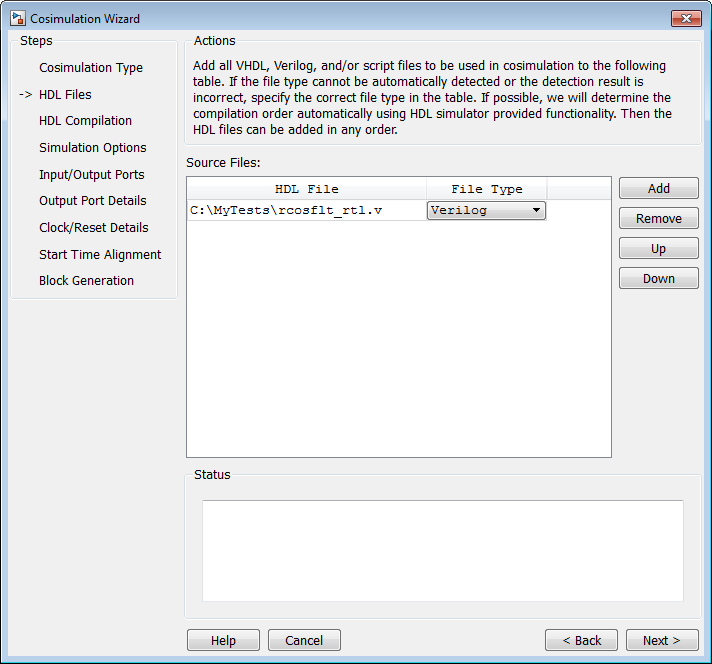2. Click to proceed to the HDL Compilation page.

Tutorial: Specify HDL Compilation Commands (Simulink)

The Cosimulation Wizard lists the default commands in the Compilation Commands window. You do not need to change these commands for this tutorial.

When you run the Cosimulation Wizard with your own code, you may add or change the compilation commands in this window. For example, you can add the -vlog01compat switch.

ModelSim users: The HDL Compilation pane will look similar to the one in this figure: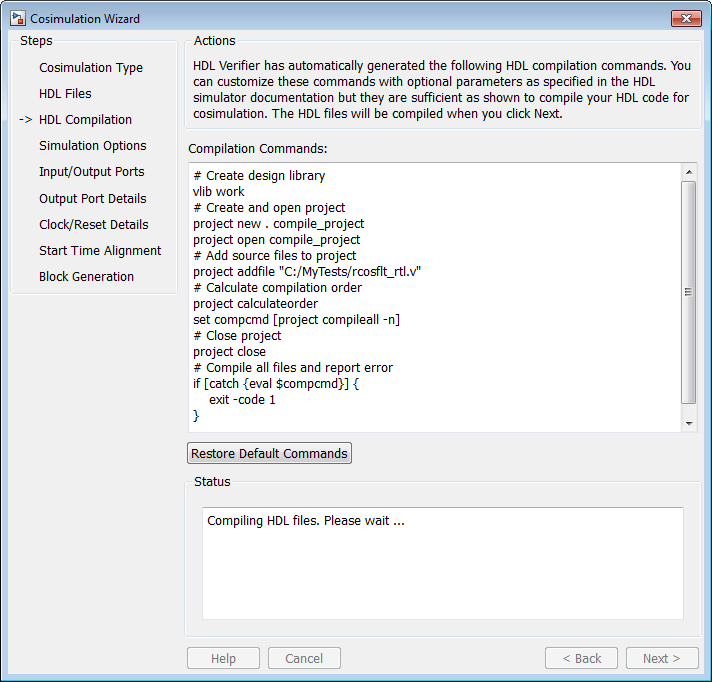Incisive users: Your HDL Compilation pane will look similar to the one in the following figure.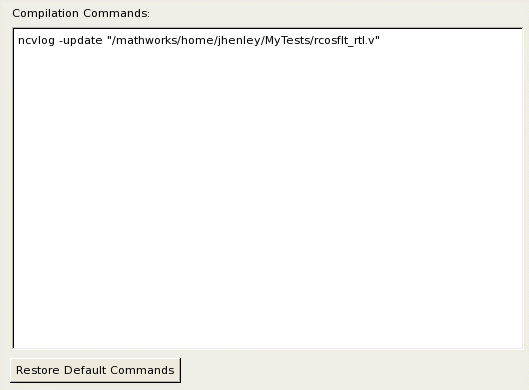Click to proceed to the HDL Modules pane.

The MATLAB console displays the compilation log. If an error occurs during compilation, that error appears in the Status area. Change whatever settings you can to remove the error before proceeding to the next step.

Tutorial: Select Simulation Options for Cosimulation (Simulink)

In the Simulation Options pane, perform the following steps:

1. Specify the name of HDL module/entity for cosimulation.

From the drop-down list, select rcosflt_rtl. This module is the Verilog/VHDL module you use for cosimulation.

If you do not see rcosflt_rtl in the drop-down list, you can enter the file name manually.

2. For Connection method, select Shared Memory if your firewall policy does not allow TCP/IP socket communication.

The simulation options now look similar to those shown in the next figure.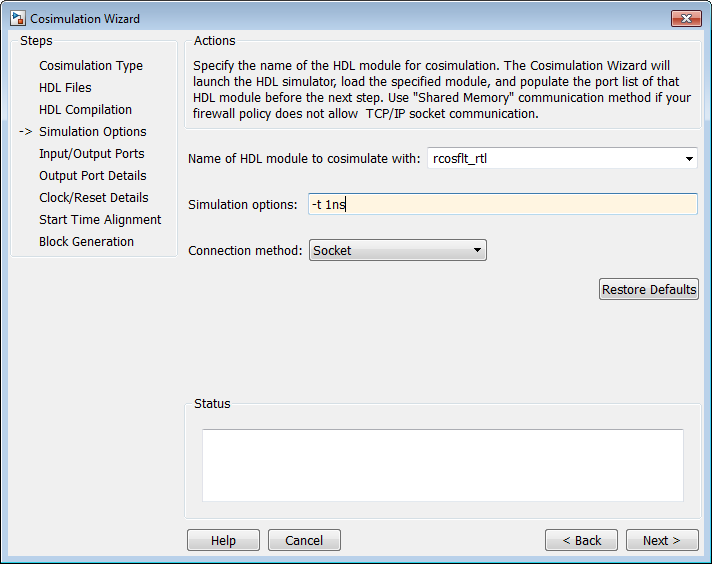Incisive users: Your HDL Module options look similar to the following figure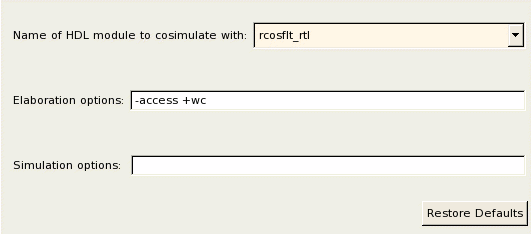3. Click to proceed to the Simulink Ports pane.

The Cosimulation Wizard launches the HDL simulator in the background console using the specified HDL module and simulation options. After the wizard launches the HDL simulator, the wizard populates the input and output ports on the Verilog/VHDL model rcosflt_rtl and displays them in the next step.

Tutorial: Specify Port Types

In this step, the Cosimulation Wizard displays two tables containing the input and output ports of rcosflt_rtl, respectively.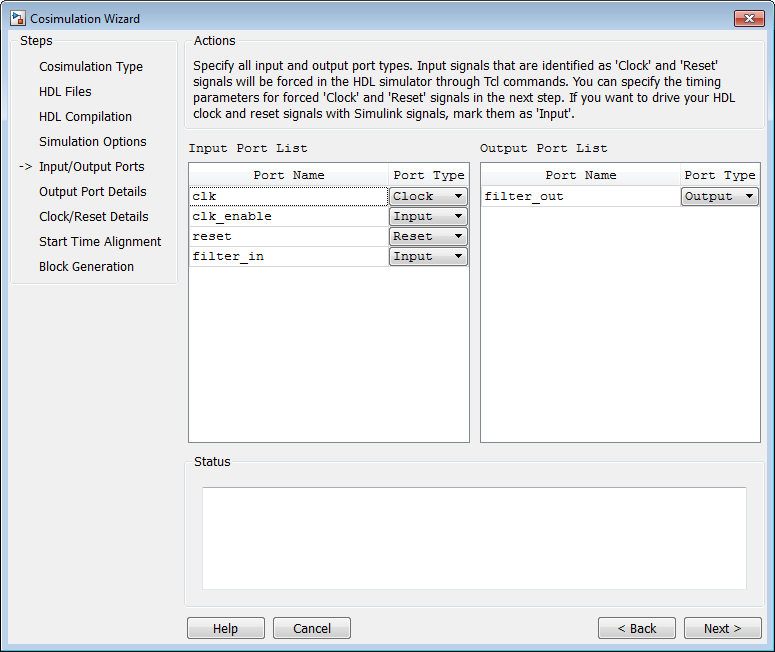The Cosimulation Wizard attempts to identify the port type for each port. If the wizard incorrectly identifies a port, you can change the port type using these tables.

• For input ports, you can select from Clock, Reset, Input, or Unused. HDL Verifier connects only the input ports marked Input to Simulink during cosimulation.

• HDL Verifier connects output ports marked Output with Simulink during cosimulation. The wizard and Simulink ignore those output ports marked Unused during cosimulation.

• You can change the parameters for signals identified as Clock and Reset at a later step.

Accept the default port types and click to proceed to the Output Port Details page.

Tutorial: Specify Output Port Details

In the Output Port Details page, perform the following steps:

1. Set the sample time of filter_out to -1 to inherit via back propagation.

2. You can see from the Verilog code that the Cosimulation Wizard represents the output in a S34,29 format. Change the following fields:

• Data Type to Fixedpoint

• Sign to Signed

• Fraction Length to 29

. Your results now look similar to the following image.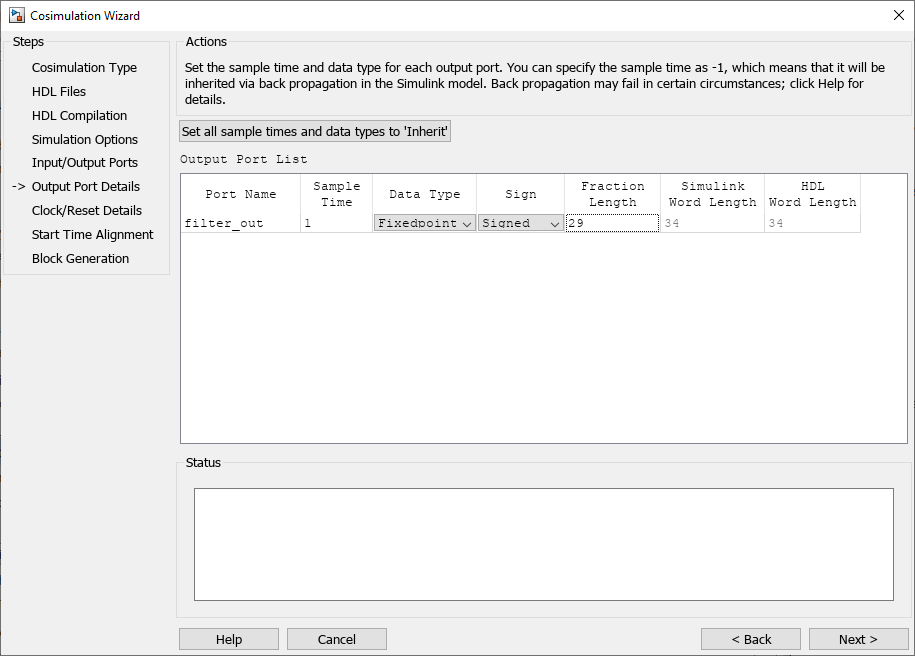3. Click to proceed to the Clock/Reset Details page.

Tutorial: Set Clock and Reset Details

For this tutorial, set the clock Period (ns) to 20. From the Verilog code, you know that the reset is synchronous and the active value is 1. You can reset the entire HDL design at time 1 ns, triggered by the rising edge of the clock. Use a duration of 15 ns for the reset signal.

In the Clock/Reset Details page, perform the following steps:

1. Set clock period to 20.

2. Leave or set active edge to Rising.

3. Leave or set reset initial value to 1.

4. Set reset signal duration to 15.

Your clock and reset are now the same as those same signals shown in the following figure.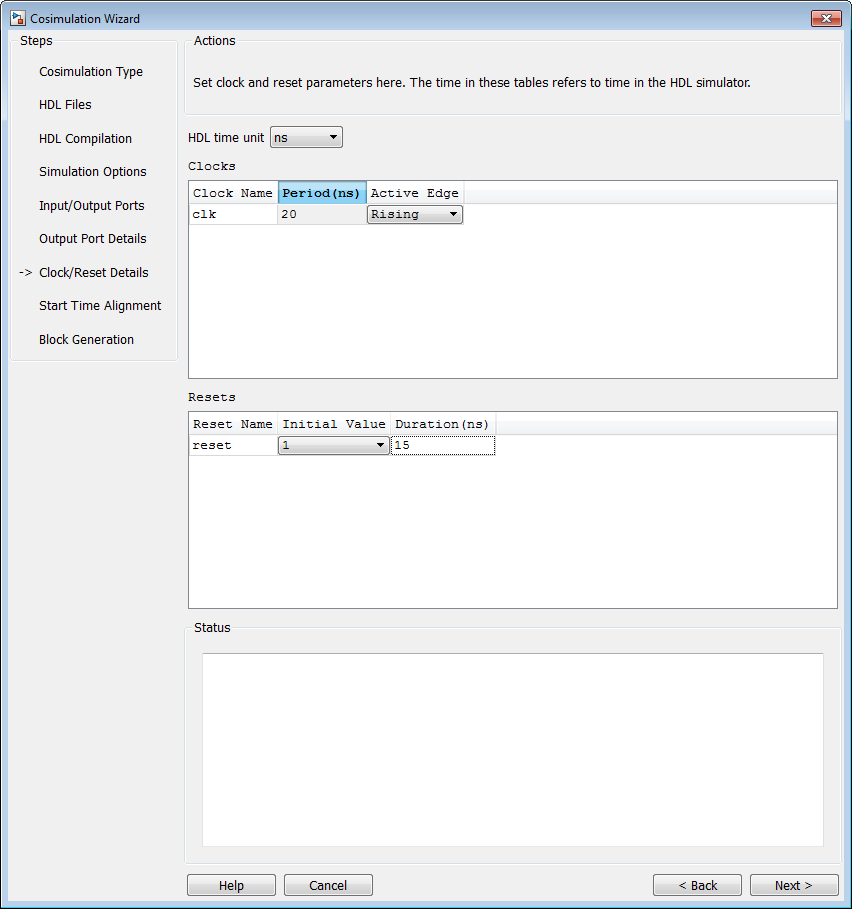5. Click to proceed to the Start Time Alignment page.

Tutorial: Confirm Start Time Alignment

The Start Time Alignment page displays a plot for the waveforms of clock and reset signals. The Cosimulation Wizard shows the HDL time to start cosimulation with a red line. The start time is also the time at which the Simulink gets the first input sample from the HDL simulator.

1. Set or confirm Start Time Alignment

The active edge of our clock is a rising edge. Thus, at time 20 ns in the HDL simulator, the registered output of the raised cosine filter is stable. No race condition exists, and the default HDL time to start cosimulation (20 ns) is what we want for this simulation. You do not need to make any changes to the start time.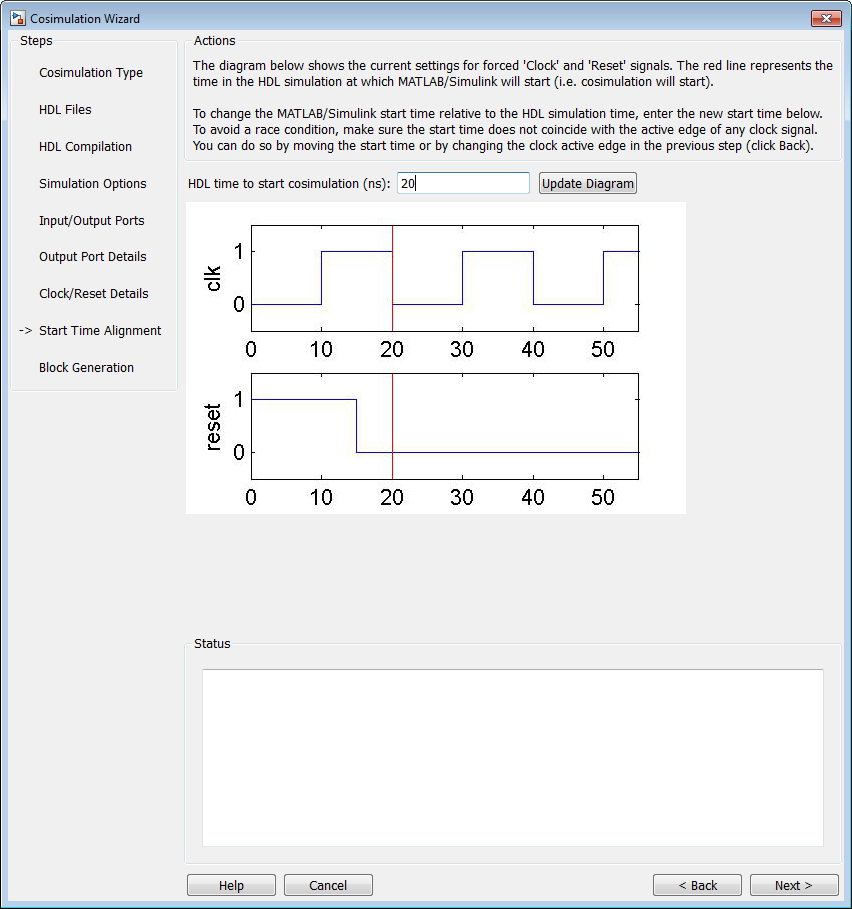2. Click to proceed to Block Generation.

Tutorial: Generate Block

Before you generate the HDL Cosimulation block, you have the option to determine the timescale before you finish the Cosimulation Wizard. Alternately, you can instruct HDL Verifier to calculate a timescale later. Timescale calculation by the verification software occurs after you connect all the input/output ports of the generated HDL Cosimulation block and start simulation.

1. Leave Automatically determine timescale at start of simulation selected (default). Later, you will have the opportunity to view the calculated timescale and change that value before you begin simulation.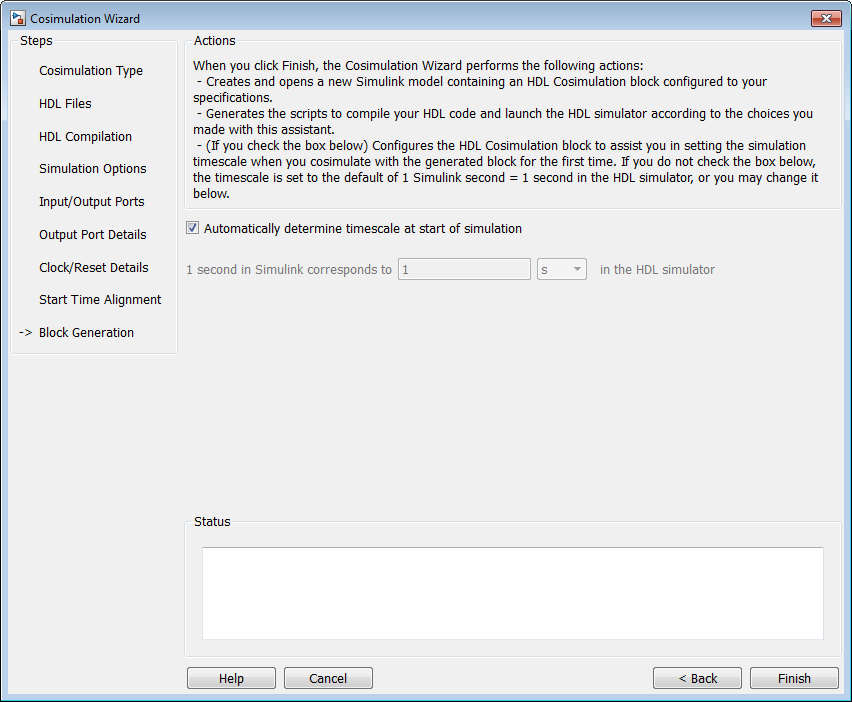2. Click to complete the Cosimulation Wizard session.

Tutorial: Create Test Bench to Verify HDL Design

For this tutorial, you do not actually create the test bench. Instead, you can find the finished model (rcosflt_tb.mdl) in the directory you created in Tutorial: Set Up Tutorial Files (Simulink).

1. After you click in the Cosimulation Wizard, Simulink creates a model and populates it with the following items:

• An HDL Cosimulation block

• A block to recompile the HDL design (contains a link to a script that is launched by double-clicking the block)

• A block to launch the HDL simulator (contains a link to a script that is launched by double-clicking the block)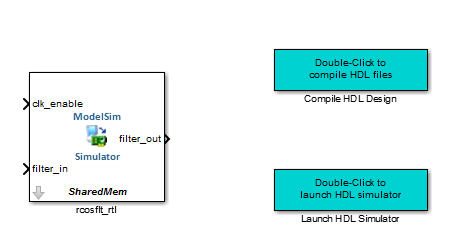Leave the model for the moment and proceed to the next step.

2. Open the file rcosflt_tb, located in the directory you created in Tutorial: Set Up Tutorial Files (Simulink).

This file contains a model of a Simulink test bench. You will use this test bench to verify the HDL design for which you just generated a corresponding HDL Cosimulation block.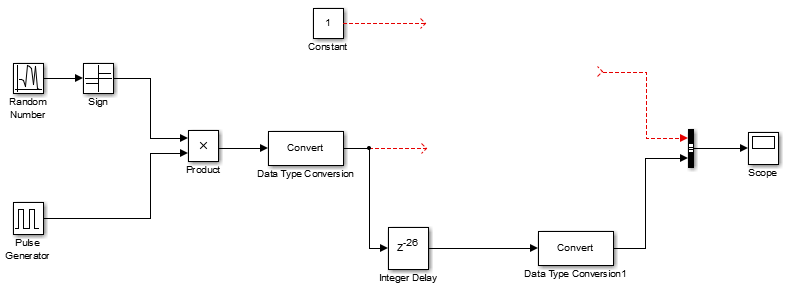3. Add the HDL Cosimulation block to the test bench model as follows:

1. Copy the HDL Cosimulation block from the newly generated model to this test bench model.

2. Place the block so that the constant and convert blocks line up as inputs to the HDL Cosimulation block and the bus lines up as output.

3. Connect the blocks in the test bench to the HDL Cosimulation block.

4. Copy the script blocks to the area below the test bench. Your model now looks similar to that in the following figure.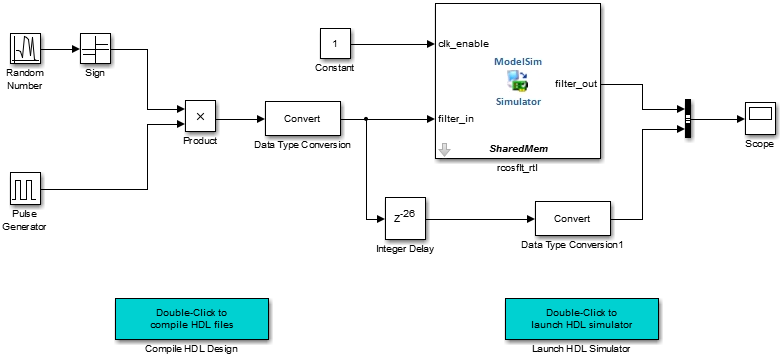5. Save the model.

Tutorial: Run Cosimulation and Verify HDL Design

1. Launch the HDL simulator by double-clicking the block labeled Launch HDL Simulator.

2. When the HDL simulator is ready, return to Simulink and start the simulation.

3. Determine timescale.

Recall that you selected Automatically determine timescale at start of simulation option on the last page of the Cosimulation Wizard. Because you did so, HDL Verifier launches the Timescale Details GUI instead of starting the simulation.

Both the HDL simulator and Simulink sample the filter_in and filter_out ports at 1 second. However, their sample time in the HDL simulator should be the same as the clock period (2 ns).

1. Change the Simulink sample time of /rcosflt_rtl/filter_in to 1 (seconds), and press Enter. The wizard then updates the table. The following figure shows the new timescale: 1 second in Simulink corresponds to 2e-008 s in the HDL simulator.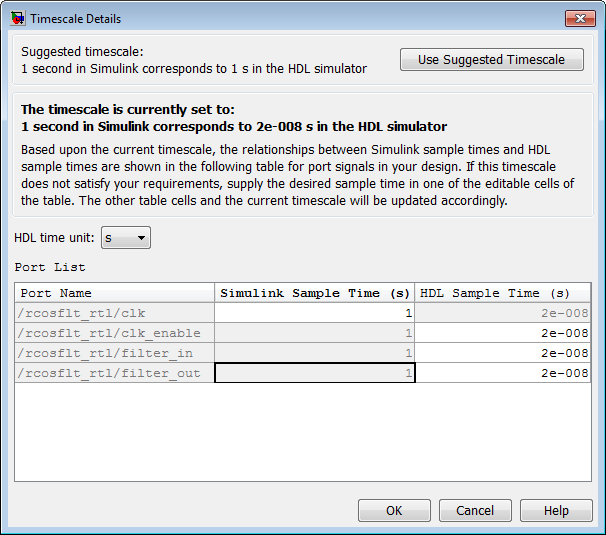2. Click to exit Timescale Details.

4. Restart simulation.

5. Verify the result from the scope in the test bench model. The scope displays both the delayed version of input to raised cosine filter and that filter's output. If you sample the output of this filter output directly, no inter-symbol-interference occurs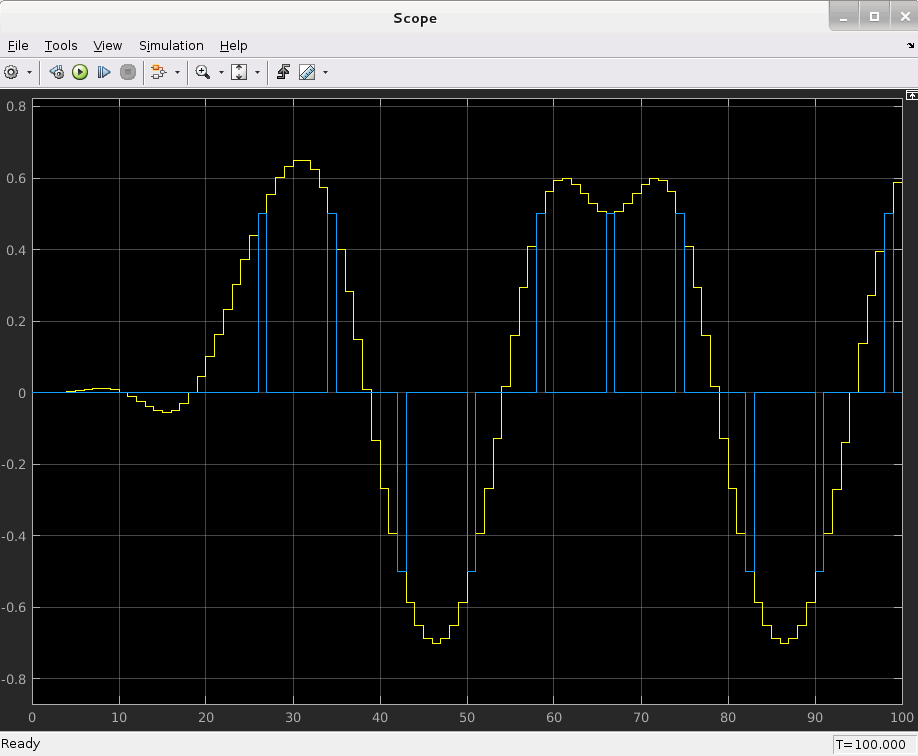This step concludes the Cosimulation Wizard for use with Simulink tutorial.

HDL Verifier Documentation평가판 신청